# Digital Communication - Sampling

Sampling is defined as, “The process of measuring the instantaneous values of continuous-time signal in a discrete form.”

Sample is a piece of data taken from the whole data which is continuous in the time domain.

When a source generates an analog signal and if that has to be digitized, having 1s and 0s i.e., High or Low, the signal has to be discretized in time. This discretization of analog signal is called as Sampling.

The following figure indicates a continuous-time signal x (t) and a sampled signal xs (t). When x (t) is multiplied by a periodic impulse train, the sampled signal xs (t) is obtained.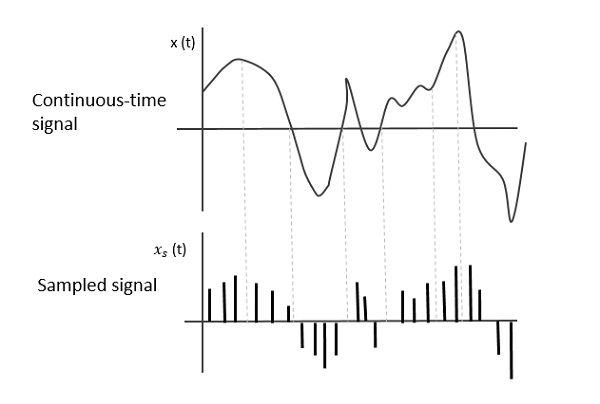## Sampling Rate

To discretize the signals, the gap between the samples should be fixed. That gap can be termed as a sampling period Ts.

$$Sampling \: Frequency = \frac{1}{T_{s}} = f_s$$

Where,

• $T_{s}$ is the sampling time

• $f_{s}$ is the sampling frequency or the sampling rate

Sampling frequency is the reciprocal of the sampling period. This sampling frequency, can be simply called as Sampling rate. The sampling rate denotes the number of samples taken per second, or for a finite set of values.

For an analog signal to be reconstructed from the digitized signal, the sampling rate should be highly considered. The rate of sampling should be such that the data in the message signal should neither be lost nor it should get over-lapped. Hence, a rate was fixed for this, called as Nyquist rate.

## Nyquist Rate

Suppose that a signal is band-limited with no frequency components higher than W Hertz. That means, W is the highest frequency. For such a signal, for effective reproduction of the original signal, the sampling rate should be twice the highest frequency.

Which means,

$$f_{S} = 2W$$

Where,

• $f_{S}$ is the sampling rate

• W is the highest frequency

This rate of sampling is called as Nyquist rate.

A theorem called, Sampling Theorem, was stated on the theory of this Nyquist rate.

## Sampling Theorem

The sampling theorem, which is also called as Nyquist theorem, delivers the theory of sufficient sample rate in terms of bandwidth for the class of functions that are bandlimited.

The sampling theorem states that, “a signal can be exactly reproduced if it is sampled at the rate fs which is greater than twice the maximum frequency W.”

To understand this sampling theorem, let us consider a band-limited signal, i.e., a signal whose value is non-zero between some –W and W Hertz.

Such a signal is represented as $x(f) = 0 for |f\lvert > W$

For the continuous-time signal x (t), the band-limited signal in frequency domain, can be represented as shown in the following figure.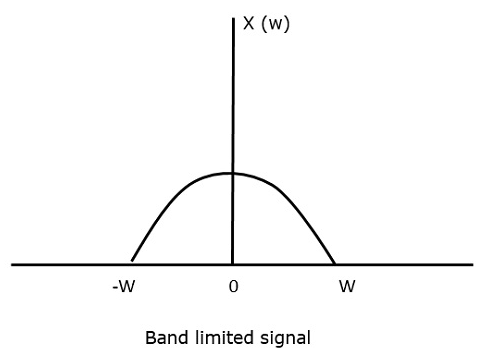We need a sampling frequency, a frequency at which there should be no loss of information, even after sampling. For this, we have the Nyquist rate that the sampling frequency should be two times the maximum frequency. It is the critical rate of sampling.

If the signal x(t) is sampled above the Nyquist rate, the original signal can be recovered, and if it is sampled below the Nyquist rate, the signal cannot be recovered.

The following figure explains a signal, if sampled at a higher rate than 2w in the frequency domain.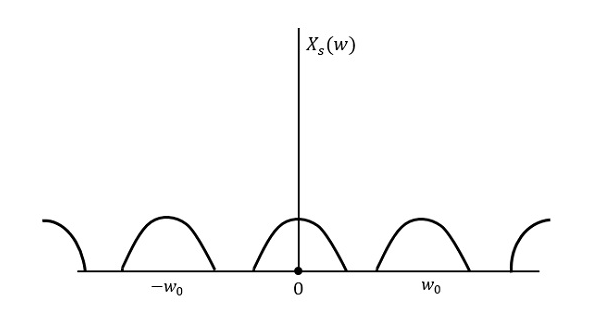The above figure shows the Fourier transform of a signal $x_{s}(t)$. Here, the information is reproduced without any loss. There is no mixing up and hence recovery is possible.

The Fourier Transform of the signal $x_{s}(t)$ is

$$X_{s}(w) = \frac{1}{T_{s}}\sum_{n = - \infty}^\infty X(w-nw_0)$$

Where $T_{s}$ = Sampling Period and $w_{0} = \frac{2 \pi}{T_s}$

Let us see what happens if the sampling rate is equal to twice the highest frequency (2W)

That means,

$$f_{s} = 2W$$

Where,

• $f_{s}$ is the sampling frequency

• W is the highest frequency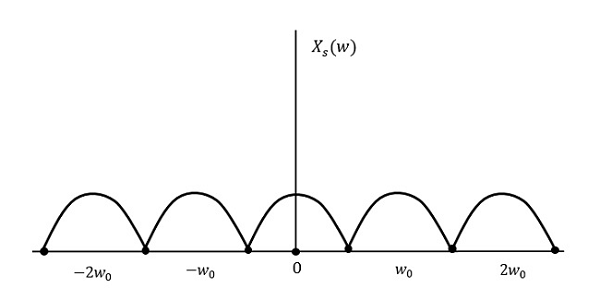The result will be as shown in the above figure. The information is replaced without any loss. Hence, this is also a good sampling rate.

Now, let us look at the condition,

$$f_{s} < 2W$$

The resultant pattern will look like the following figure.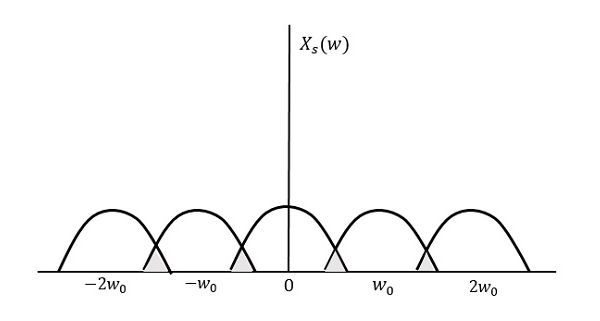We can observe from the above pattern that the over-lapping of information is done, which leads to mixing up and loss of information. This unwanted phenomenon of over-lapping is called as Aliasing.

## Aliasing

Aliasing can be referred to as “the phenomenon of a high-frequency component in the spectrum of a signal, taking on the identity of a low-frequency component in the spectrum of its sampled version.”

The corrective measures taken to reduce the effect of Aliasing are −

• In the transmitter section of PCM, a low pass anti-aliasing filter is employed, before the sampler, to eliminate the high frequency components, which are unwanted.

• The signal which is sampled after filtering, is sampled at a rate slightly higher than the Nyquist rate.

This choice of having the sampling rate higher than Nyquist rate, also helps in the easier design of the reconstruction filter at the receiver.

## Scope of Fourier Transform

It is generally observed that, we seek the help of Fourier series and Fourier transforms in analyzing the signals and also in proving theorems. It is because −

• The Fourier Transform is the extension of Fourier series for non-periodic signals.

• Fourier transform is a powerful mathematical tool which helps to view the signals in different domains and helps to analyze the signals easily.

• Any signal can be decomposed in terms of sum of sines and cosines using this Fourier transform.

In the next chapter, let us discuss about the concept of Quantization.